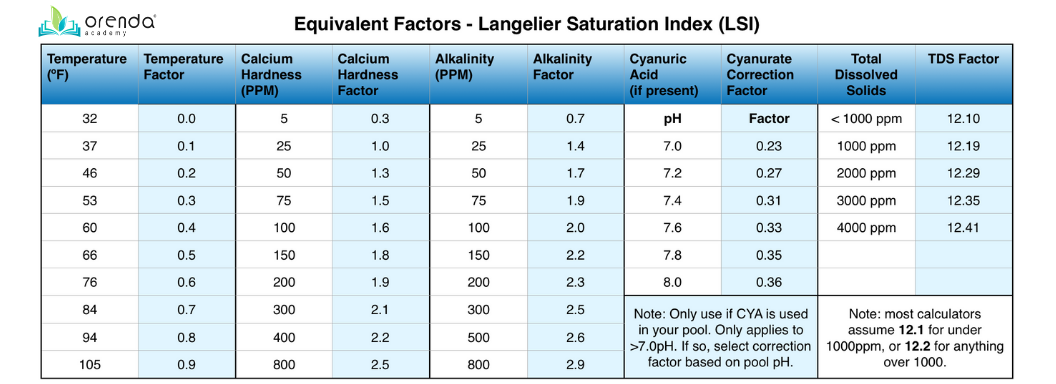The LSI equation calls for the carbonate alkalinity of pool water...but most pool test kits just measure total alkalinity. What's the difference between carbonate alkalinity and total alkalinity?

# Carbonate Alkalinity can be calculated from Total Alkalinity...if the pH is known

Let's start with a quick recap of what alkalinity actually is. Total alkalinity is a measurement of all dissolved alkaline substances in the water. Primarily, these are bicarbonates, carbonates, and hydroxidesEach of these types of alkaline products can be calculated through scientific formulas, assuming you know the pH of the water. In other words, if you test the total alkalinity of the water, and test the pH, you can then derive the carbonate alkalinity with a scientific formula. But it can get complicated.

It is important to know, because the Langelier Saturation Index (LSI) calls for the carbonate alkalinity in its equation. Fortunately for you, our LSI calculator app does the equation for you. So all you need to do is enter the total alkalinity, CYA and pH...our algorithm does the rest. The calculator won't show you the carbonate alkalinity, but it will factor it into the LSI equation automatically. But just in case you are wondering what the carbonate alkalinity formula looks like:

Carbonate Alkalinity (CaCO3 mg/L) = 100,000 [K2 (HCO3-)(10pH)]

For swimming pools it's much easier. The swimming pool adaptation of the LSI actually calls for a "correction factor" to find carbonate alkalinity by subtracting cyanurate alkalinity from total alkalinity. This correction is why we need to know our cyanuric acid level in ppm, and our pH. The chart below shows different correction factors depending on the pH, which all plug in nicely into the formula. The rough rule of thumb is to subtract 1/3 of your CYA ppm from your TA ppm. But that's just an estimate.

Increased stabilizer means a lowered LSI, because it "corrects" total alkalinity down to just carbonate alkalinity. We know, it's confusing. We promise we didn't come up with the formula. It is what it is. Here's the table of LSI factors:Notice the correction factor that Cyanuric Acid has. It "corrects" total alkalinity down to just carbonate alkalinity.

## It's easy to confuse alkaline names

Part of the reason the topic of alkalinity is so misunderstood is because of the word itself. On the pH scale, below neutral seven (7) is acidic. Above seven is basic, or alkaline. Dissolving alkaline products in the pool raises both your pH and total alkalinity.

• Carbonates -- like calcium carbonate (CaCO3), or sodium carbonate (Na2CO3) "soda ash"
• Bicarbonates -- like sodium bicarbonate (NaHCO3) "sodium bicarb"
• Hydroxides -- like sodium hydroxide (NaOH) "caustic soda" or "lye", or calcium hydroxide (Ca(OH)2) "lime".

That said, the pH of these products--while all alkaline--are not equal. In a 20,000 gallon backyard pool, for example, you can raise the alkalinity 10ppm with either 1.77 pounds of soda ash, or 2.8 pounds of sodium bicarb. Soda ash would be boosting the carbonate alkalinity, but not hydroxides or bicarbonates. The pH would be different two, since the substances have slightly different pH values. As you can see, it's easy to confuse pH and alkalinity. We're trying our best to make the distinction clear and understandable.

The distinction between these forms of alkalinity are determined by the pH. The higher the pH, the less hydrogen present, which changes the species of alkalinity. Here's a reference chart:Below 4.3 pH, there is no alkalinity...just carbonic acid with 2 Hydrogens. Then Bicarbonate alkalinity shows up, but has only 1 Hydrogen. Then Carbonate alkalinity with no Hydrogen.

What's important here is not the math or the complex science behind all this. If you wanted to know all that, we encourage you to do the research on your own, and you can start here, here and here. The important thing is knowing what alkalinity is, and why it's important. Alkalinity's primary function is to buffer the pH in the water; in other words, help water resist change in pH. Without the right amount of alkaline substances in the water, the pH will tend to fluctuate. Just ask any pool manager.## Stabilize pH and LSI with calcium and alkalinity

We at Orenda believe water balance should be a top priority in pool management, right up there with sanitization. Yes, even before range chemistry. Why? Because without proper water balance, range chemistry can get out of control fast, and chlorine can be largely ineffective. Stabilizing pH, for example, requires a good amount of calcium and alkalinity in the water.  We recommend using our dosing calculator to find what numbers work for your pool.  Here are Orenda's recommendations for the low/high of each factor, provided LSI balance is maintained:

We have found so many problems go away when the pool chemistry is LSI balanced. The not only are etching and scale less likely to happen, but less chemicals are required to keep the pH balanced. Wouldn't it be great if you weren't constantly battling pH with acid and alkaline products like sodium bicarb or soda ash? How much less would you spend in pH adjusting chemicals if your LSI was balanced each and every week? We encourage you to try it and see how it works for you...you'll be glad you did.

# Summary

Unlike pH, which is a scale to measure on, total alkalinity is the amount of dissolved alkaline substances in water. It's measured in parts-per-million. There are three types of alkaline products that make up Total alkalinity: carbonates, bicarbonates and hydroxides (examples listed above). To calculate the carbonate alkalinity, there is a formula, provided the pH is known. But no worries...the Orenda App dosing calculator has you covered. Our LSI calculator automatically factors in the formula to derive the carbonate alkalinity from the total, so you don't have to.  Enjoy managing balanced water, because it's a whole lot easier than not.